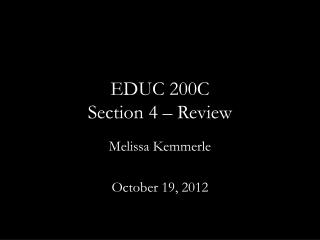Download PresentationEDUC 200C Section 4 – Review

# EDUC 200C Section 4 – Review

Download Presentation## EDUC 200C Section 4 – Review

- - - - - - - - - - - - - - - - - - - - - - - - - - - E N D - - - - - - - - - - - - - - - - - - - - - - - - - - -
##### Presentation Transcript

1. EDUC 200CSection 4 – Review Melissa Kemmerle October 19, 2012

2. Goals • Review regression and measures of fit • Review Spearman correlation and relationship to Pearson correlation • Talk briefly about normal distributions • Quick review of everything—midterm next Wednesday • Questions

3. Regression • Use regression to predict how one variable changes in response to another variable. • Prediction line is calculated by minimizing the total squared difference between the line representing our prediction and the actual data

4. Regression line notation and formulas Y’ = bYXX + aYX Regression line slope: bYX = rYX (σy / σx) Regression line intercept: aYX = Y - bYXX

5. How do we know if we’ve explained the data well? • Standard error is the same as standard deviation except that we look at deviation from the prediction rather than deviation from the mean

6. Extreme examples • Same Y data with differing relationships to X

7. Standard Deviation is relative to the mean • Since the Y data is identical in both graphs, the total variance of Y is also identical

8. Standard Error is relative to the prediction • The different relationships of Y to X is reflected by how close predicted values of Y are to actual values

9. Explained vs. Unexplained variance

10. How much variance have we explained? • You can crudely think of the error variance as how much variance in Y is “left over” after accounting for X • Knowing X gets us close, but probably not all the way, to knowing Y • gives the percent of total variance in Y that we have not explained with X • thus gives percent of variance in Y explained by X • Conveniently, this is equal to • Can also think of this as the percent of shared variance between X an Y

11. Stata… Error variance, sY’2 R-squared, rYX2 . Reg Y X Source | SS df MS Number of obs = 50 -------------+------------------------------ F( 1, 48) = 4312.81 Model | 3.92107903 1 3.92107903 Prob > F = 0.0000 Residual | .043640216 48 .000909171 R-squared = 0.9890 -------------+------------------------------ Adj R-squared = 0.9888 Total | 3.96471925 49 .080912638 Root MSE = .03015 ------------------------------------------------------------------------------ Y | Coef. Std. Err. t P>|t| [95% Conf. Interval] -------------+---------------------------------------------------------------- X | .0194055 .0002955 65.67 0.000 .0188114 .0199996 _cons | .0418653 .008658 4.84 0.000 .0244573 .0592733 ------------------------------------------------------------------------------ Note that these values have bias corrections that make them more like s than σ Total variance, sY2 Standard error, sY’

12. Spearman Correlation • Identical to Pearson correlation except that we are specifically dealing with rank-order data rather than continuous data • Gives a measure of the relationship of the relative ranks rather than relative values Where D is the difference in ranks, rather than difference if values, for the same observation

13. Spearman vs. Pearson • When using rank-order data, using the Spearman formula and the Pearson formula will give you identical results • The Spearman rank-order correlation coefficient is usually different from the Pearson r correlation coefficient if the Pearson r is calculated using untransformed data (i.e. not rank-order data) • Consider the case where you keep increasing the highest value of one of the variables of interest—this will affect the Pearson correlation, but not the Spearman correlation

14. The Normal Curve

15. The null hypothesis • Example: A study compares the results of a new reading program for middle school students. In this study, 36 students received the experimental reading program • Each student’s reading score was measured before and after the program. The variable of interest was score change • Score change was positive if a student’s score improved and negative if the score got worse • What is our null hypothesis?

16. Hypothesis testing vocabulary Null Hypothesis: A hypothesis to be tested. • Use the symbol H0 (e.g. H0 : μ=0) Alternative Hypothesis: A hypothesis that represents the opposite of the null hypothesis • One or the other must be true, there can be no third option • Use the symbol HA or H1 (e.g. HA : μ≠0) Hypothesis Test: The test of whether the null hypothesis (H0) should be rejected in favor of the alternative hypothesis.

17. Questions so far?

18. Review of Everything • Measures of central tendency • Mean: • Median: value greater than 50% of all other observations • Mode: most common value

19. Review of Everything • Measures of Spread • Population variance, σ2: • (Unbiased) sample variance, s2: • Population standard error, σ: • (Unbiased) sample standard error, s:

20. Review of Everything • Z – scores • Data transformation to give data a mean on 0 and a standard deviation of 1

21. Review of Everything • Correlation • Pearson r correlation coefficient • Z-score difference formula • Z-score product formula • Raw score formula

22. Review of Everything • Correlation • Spearman rank-order correlation coefficient

23. Review of Everything • Regression • Predict Y from X: • Error (or residual):

24. Review of Everything • Regression • Standard error: • R-squared: R2 gives us the percent of variance in Y explained by X. This is sometimes called percent of shared variance.

25. Questions?